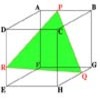#### You may also like### Cut Cube

Find the shape and symmetries of the two pieces of this cut cube.### Maximum Scattering

Your data is a set of positive numbers. What is the maximum value that the standard deviation can take?

# Walls

##### Age 16 to 18 Challenge Level:

If a plane contains a variable point $P$ with position vector ${\bf r}$, and if ${\bf n}$ is a unit vector perpendicular to the plane, then consider the right angled triangle $OPN$, where $N$ is the foot of the perpendicular from the origin $O$ to the plane and $ON=d$ is the perpendicular distance from the origin to the plane. Then $ON = OP \cos \angle PON$ which is given by the scalar product ${\bf r.n}$ so the equation of the plane is given by ${\bf r.n}= d$.

In Cartesian form ${\bf r}=(x,y,z)$ and ${\bf n} = (a,b,c)$ so ${\bf r.n}= d$ translates to $ax+by+cz=d$.